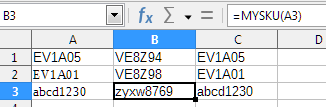# Libre Office Excel supports both TEXTJOIN and SEQUENCE?

How can I make functions to work? Spreadsheet in attach.nekbv.xlsx

Only TEXTJOIN() is supported, SEQUENCE() or any other array spilling function is not, hence you see its raw `_xlfn.sequence` name and opening the formula cell in the Function Wizard (Ctrl+F2) on the Structure tab it’s marked with a red dot crossed.

Good to know.
Anyway, what I want is:

I have about 22000 lines with SKU product numbers from the manufacturer. I want to sell their products with my own SKU numbers, but I want my SKU numbers to be based on their SKU number. How can I do this?
Exemple in attach.EXEMPLELIBRE2.xlsx

Let E2 be an input of their SKU, then use VLOOKUP() to lookup your SKU, so in F2 have

``=VLOOKUP(E2;\$A\$2:\$B\$7;2;0)``

Something is not ok…
So the ideea is:

Manufacturer SKU * TRANSFORM TO * My SKU
EV1A05 * E=V V=E 1=8 A=Z 0=9 5=4 * VE8Z94
EV1A01 * E=V V=E 1=8 A=Z 0=9 1=8 * VE8Z98

If manufacturer SKU is abcd1230 I want automaticaly my SKU to become zyxw8769.
In Clollumn A will be MY SKU, in collumn B will be the Manufacturer SKU.

A=Z B=Y C=X D=W E=V F=U G=T H=S I=R J=Q K=P L=O M=N N=M O=L P=K Q=J R=I S=H T=G U=F V=E W=D X=C Y=B Z=A
0=9 1=8 2=7 3=6 4=5 5=4 6=3 7=2 8=1 9=0

Since we know that `Asc("A") + Asc("Z") = 155` and `Asc("a") + Asc("z") = 219`, the solution is very simple:

``````Function MYSKU(sText As String) As String
Dim i As Integer
Dim ch As String
Dim sResult As String
For i = 1 To Len(sText)
ch = Mid(sText, i, 1)
Select Case ch
Case "A" To "Z"
sResult = sResult & Chr(155 - Asc(ch) )
Case "a" To "z"
sResult = sResult & Chr(219 - Asc(ch))
Case "0" To "9"
sResult = sResult & Trim(9-Val(ch))
Case Else
sResult = sResult & ch
End Select
Next i
MYSKU = sResult
End Function
``````The code prove to be simple because the encryption (transcoding) method described in the comment turned out to be symmetric - if you reapply the function to the conversion result, you get the original string.

In case the recoding is performed using unordered dictionaries, the code will not be much more complicated.

``````Function aCrypto(sText As String, bDirection As Boolean) As String
Const SOURCE_CHARS = "ABCDEFGHIJKLMNOPQRSTUVWXYZabcdefghijklmnopqrstuvwxyz0123456789"
Const CRYPTO_CHARS = "EQSWCYKPORBDMAXFVJHTGLZUNIfmwngvhpzuaosirlctbyxkqdej9765328410"
Dim i As Integer, iPos As Integer
Dim ch As String
Dim sResult As String
For i = 1 To Len(sText)
ch = Mid(sText, i, 1)
If bDirection Then
iPos= InStr(SOURCE_CHARS, ch)
If iPos = 0 Then
sResult = sResult & ch
Else
sResult = sResult & Mid(CRYPTO_CHARS, iPos, 1)
EndIf
Else
iPos= InStr(CRYPTO_CHARS, ch)
If iPos = 0 Then
sResult = sResult & ch
Else
sResult = sResult & Mid(SOURCE_CHARS, iPos, 1)
EndIf
EndIf
Next i
aCrypto = sResult
End Function
``````

To encode the manufacturer’s code into your own code, you need to execute `=ACRYPTO(B2; 1)` and for reverse transcoding you need to do `=ACRYPTO(B2; 0)`

In order not to use the second parameter, you can make two simple wrapper functions:

``````Function mfg_to_my(sText As String) As String
mfg_to_my = aCrypto(sText, TRUE)
End Function

Function my_to_mfg(sText As String) As String
my_to_mfg = aCrypto(sText, FALSE)
End Function
``````

Then for encoding, you can write `=MFG_TO_MY(B2)`, and for decoding `=MY_TO_MFG(C2)`

It is possible to write two separate functions at once instead of the aCrypto () function, but that would mean that the “transcoding dictionaries” would also have to be inserted twice in the code, and this can lead to confusion if these dictionaries need to be changed.

im really trying to learn how to use LibreOffice, im a beginner into this.
How Im gonna insert youre example in my LibreOffice Excel WorkFile?

This should not be inserted into the Excel file, it is better to put it into your Standard macro library. It is difficult to explain in a few words. For now, watch this video - here it is shown how to install in your LibreOffice a macro, the code of which is published somewhere on the Internet. There are a lot of short videos on YouTube that clearly show the techniques for working with an office suite. Then read this text - I can’t write it in more detail.